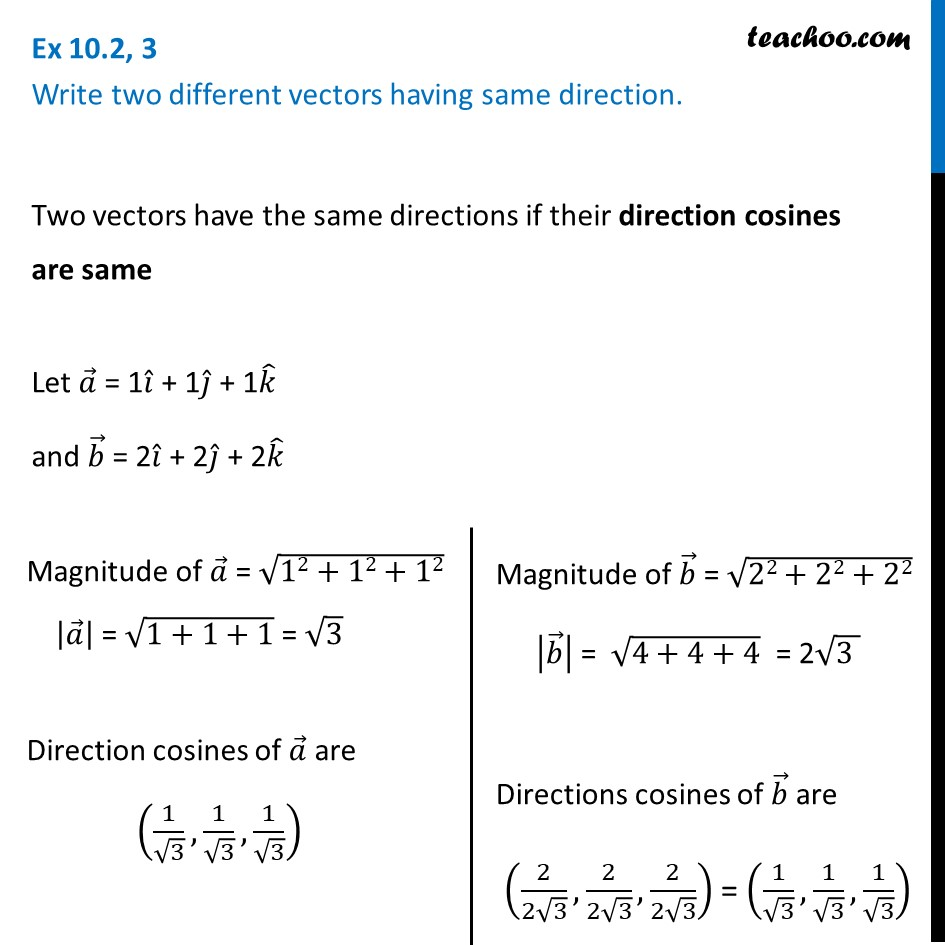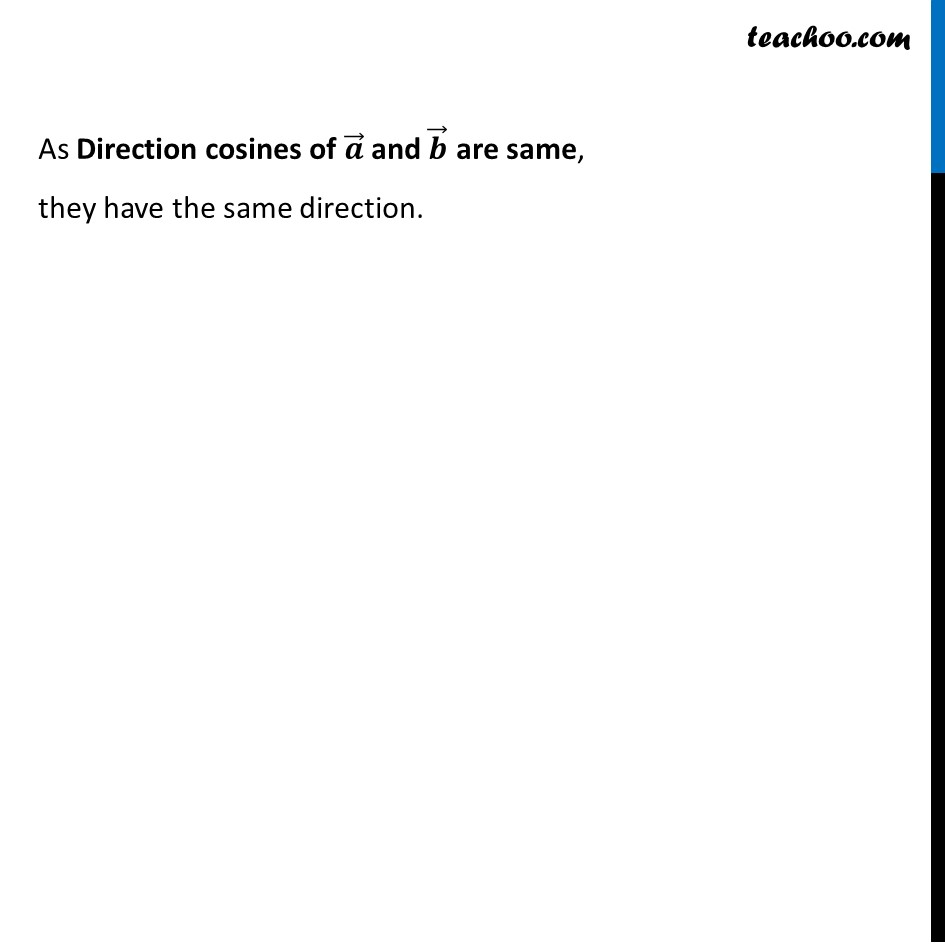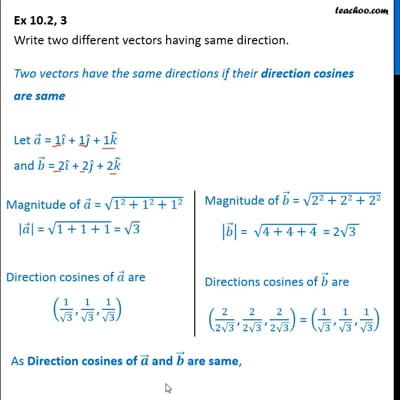Ex 10.2

Chapter 10 Class 12 Vector Algebra
Serial order wiseThis video is only available for Teachoo black users

Introducing your new favourite teacher - Teachoo Black, at only ₹83 per month

### Transcript

Ex 10.2, 3 Write two different vectors having same direction.Two vectors have the same directions if their direction cosines are same Let 𝑎 ⃗ = 1𝑖 ̂ + 1𝑗 ̂ + 1𝑘 ̂ and 𝑏 ⃗ = 2𝑖 ̂ + 2𝑗 ̂ + 2𝑘 ̂ Magnitude of 𝑎 ⃗ = √(12+12+12) |𝑎 ⃗ | = √(1+1+1) = √3 Direction cosines of 𝑎 ⃗ are (1/√3,1/√3,1/√3) Magnitude of 𝑏 ⃗ = √(22+22+22) |𝑏 ⃗ | = √(4+4+4) = 2√(3 ) Directions cosines of 𝑏 ⃗ are (2/(2√3),2/(2√3),2/(2√3)) = (1/√3,1/√3,1/√3) As Direction cosines of 𝒂 ⃗ and 𝒃 ⃗ are same, they have the same direction.